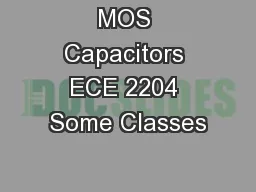# MOS Capacitors ECE 2204 Some Classes2018-09-22 3K 3 0 0

## MOS Capacitors ECE 2204 Some Classes - Description

of Field Effect Transistors. M. etal-. O. xide-. S. emiconductor Field Effect Transistor. MOSFET, which . will be the type that we will study in this course.. Me. tal-. S. emiconductor Field Effect Transistor. ID: 674952 Download Presentation

Embed code:

## MOS Capacitors ECE 2204 Some Classes

Download Presentation - The PPT/PDF document "MOS Capacitors ECE 2204 Some Classes" is the property of its rightful owner. Permission is granted to download and print the materials on this web site for personal, non-commercial use only, and to display it on your personal computer provided you do not modify the materials and that you retain all copyright notices contained in the materials. By downloading content from our website, you accept the terms of this agreement.

### Presentations text content in MOS Capacitors ECE 2204 Some Classes

Slide1

MOS Capacitors

ECE 2204

Slide2

Some Classes

of Field Effect Transistors

M

etal-

O

xide-

S

emiconductor Field Effect Transistor

MOSFET, which

will be the type that we will study in this course.

Me

tal-

S

emiconductor Field Effect Transistor

MESFET, typically fabricated with III-V semiconductors

J

unction Field Effect Transistor

JFET, resilient to electrostatic discharge (ESD)

H

igh

E

lectron

M

obility

T

ransistor or

Mo

dulation

D

oped Field Effect Transistor

HEMT or

MODFET

, typically fabricated with III-V

semiconductors

F

ast

Re

verse/

F

ast

R

ecovery

E

pitaxial

D

iode

FREDFET

DNA Field Effect Transistor

The conduction path is through a strand of DNA

Slide3

Field Effect Transistors

A voltage is applied to the gate of the transistor, which produces an electric field within the semiconductor.

Typically, the gate current is zero.

The

conductivity (or resistivity) of the path between two contacts, the source and the drain, is altered by the voltage applied to the

gate.

Device is also known as a voltage controlled resistor.

Slide4

MOS Capacitor

The operation of a metal-oxide-semiconductor is used to explain the operation of the MOSFET.

In

a MOSFET, the channel in the semiconductor, the oxide, and the gate

metalization

forms a MOS capacitor.

The structure looks like a parallel plate capacitor where one of the plates is the

semiconductor

and the other is the gate metalization. The insulator between the parallel plates is the oxide.

Semiconductor

Oxide

Slide5

MOS Capacitor

e

ox

t

ox

e

OX

=

e

ox

e

o

where

e

ox

is the relative dielectric constant of the oxide.

Slide6

Operation of a MOS Capacitor

In the following analysis, it is assumed that the channel in the semiconductor is lightly doped with acceptors (i.e., p type).

There are three regions of operation

Accumulation (in this case, V

G

< 0 V)

Depletion

(in this case, 0 V ≤

VG ≤ VTN)Inversion (in this case, VG ≥ VTN)

Slide7

Accumulation (V

G

< 0 V)

An electric field E is induced by the applied voltage V

G

.

More holes are at the oxide-semiconductor interface than expected from the concentration of acceptors.

Holes are attracted to interface by the negative gate voltage. Electrons are repelled towards the body contact.

Slide8

Depletion

(0 V < V

G

≤ V

TN

)

The induced electric

field E

causes the concentration of holes at the oxide-semiconductor interface to be smaller than the acceptor concentration and the electron concentration to be greater than expected.

Slide9

Depletion

M

ode Capacitance

The unscreened acceptors and a higher than expected electron concentration at the oxide semiconductor interface induce an electric field in the semiconductor, producing a depletion region to form

.

Slide10

Inversion (V

G

V

TN

)

The gate voltage is large enough that the concentration of electrons at the oxide-semiconductor interface is greater than the concentration of holes.

The type of the semiconductor has effectively been converted from p-type to n-type. The voltage when n = p at the oxide-semiconductor interface is called the threshold voltage, V

TN.

Slide11

Inversion M

ode Capacitance

A depletion

region

still exists in the semiconductor, but is now located between the inverted

(n-type)

region and the remaining p-type semiconductor.

The thickness of the depletion region W is constant, even if VG

increases so the magnitude of the MOS capacitance is constant.

Slide12

MOS Capacitance

Is largest in the accumulation mode when V

G

≤ 0 V

Is smallest in the inversion mode

when V

G ≥ V

TNVaries with V

G in the depletion mode

Slide13

Questions

If the acceptor concentration is increased, will be threshold voltage have to increase or decrease?

Do you expect the threshold voltage to increase or decrease as the temperature of the MOS capacitor is increased?

Can you explain how the electron and hole concentration varies with V

G

if the semiconductor is initially n-type instead of p-type?

Slide14